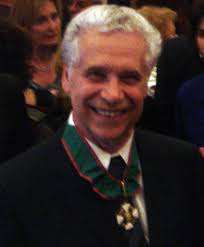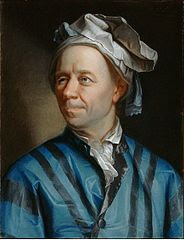## Breaking News

### The Birth of Strings

The story goes back to late 1968 AD; when an Italian physicist named “Gabriel Veneziano” was searching for a set of mathematical equation that can explain the strong nuclear force.Gabriel Veneziano

Strong nuclear force is one of the four fundamental forces that hold protons and neutrons together inside the atom.

Veneziano finally found the solution in a 200 year old equation written by Leonard Euler and this equation tells about the gamma function.Leonard Euler
The message then spread out and a young American physicist named Leonard Susskind finally figures out something fundamental out of this gamma function. He found though the equation describes the strong nuclear force, it also describes the internal structure of the particles which is not just a point but like a string.Leonard Susskind

Yes, it is a string just like a closed rubber band or an open one ; which could vibrate and it is present inside the particles like quarks , electrons etc.
This is the birth of “THE STRING THEORY”

### Problems associated with string theory

But in its early stage, string theory was associated with a lot of problems. Some of them are –:
• It describes a particle named “Tychon” which could travel faster than the speed of light.
• The string do not require 3 dimensional space as we know rather, it defined itself in 10 dimensions and those are -: 3 regular dimensions , One dimension for time and rest are special dimensions
• String theory also has mass less particles, i.e., particles without any mass.
• It contains mathematical anomalies.
Mathematical anomalies can be understood means getting different values for the same situation like for example if in a certain processing voltage requirement is 230 volts then it does not matter in how many different ways we calculate voltage as in all the case we will find the same 230 volts but mathematical anomalies will occur when we will get different values of the voltage by different ways of finding it for the same condition.
Moreover; this was the era when particle physics is at its apex position and new particles were discovered in every other day by means of particle accelerators. Physicist used to study these new particles and arranging them into certain groups to find the building blocks of the universe and later they came up with “THE STANDARD MODEL”. In this situation, theory like string theory faced ignorance.

### Revolution came in String theory

In 1984 AD; Michael Green & John H. Schwarz were able to figure out the mathematical anomalies present in the string theory and from this moment; the string theory got a thrust and became popular in the field of physics.

### What actually the string theory tells us?

In a simple language; the string theory tells the following -:

#### The fundamental building block of each matter is strings.

As we all know each matter around us are made up of atoms; which in turn made up of three fundamental particles called electrons, protons and neutrons. But the story does not ends here ; particles like proton and neutrons are still made up of even more small particles called as “quarks” and as per the string theory ; these tiny matters like quarks, electrons etc. are made by the strings. So, it is the strings which makes everything and hence can be called as the building block of the universe.

#### Strings vibrate to create fundamental particles like electrons, quarks etc.

This can be understood by taking the example of a guitar ; as in guitar the vibration of certain strings in a certain way creates a particular node ; similarly the tiny stings vibrate & wiggle in different patterns to create different fundamental particles having different properties such as electrons , quarks etc.

#### String theory has potential to give “The theory of everything”

The TOE (Theory of everything) means a theory that could explain both relativistic physics (The laws that govern matters in a larger scale like stars, planets, galaxies, black holes etc and interactions between them) and quantum mechanics (The set of laws that are completely different and governs matter in a very smaller scale like subatomic particles, quarks etc.) in a single equation.

The fundamental problem of physics is -:

It is broadly divided in two parts as said above and the laws for the one part don’t hold good for the other and vice-versa.

So, the ultimate aim of physics is nothing but to unify these two branches and it is the string theory can act as the bridge for this.

Thank you
Quantum X

Credits-:

For the image of Jhon H. Scwarhz -: theory.caltech.edu
for the image of Michael Green -: icts.res.in